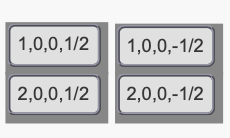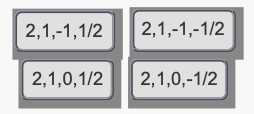# Problem: Part B. Identify the sets of quantum numbers that describe all the electrons in the ground state of a neutral beryllium atom, Be. Each set is ordered (n, ℓ, mℓ , ms).Every electron in an atom is described by a unique set of four quantum numbers: n, ℓ, mℓ, and ms. The principal quantum number, nn, identifies the shell in which the electron is found. The angular momentum quantum number, ℓ, indicates the kind of subshell. The magnetic quantum number, mℓ , distinguishes the orbitals within a subshell. The spin quantum number, msm_s, specifies the electron spin.

###### FREE Expert SolutionView Complete Written Solution
###### Problem Details

Part B. Identify the sets of quantum numbers that describe all the electrons in the ground state of a neutral beryllium atom, Be. Each set is ordered (n, ℓ, mℓ , ms).Every electron in an atom is described by a unique set of four quantum numbers: n, ℓ, m, and ms. The principal quantum number, nn, identifies the shell in which the electron is found. The angular momentum quantum number, ℓ, indicates the kind of subshell. The magnetic quantum number, m , distinguishes the orbitals within a subshell. The spin quantum number, msm_s, specifies the electron spin.